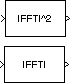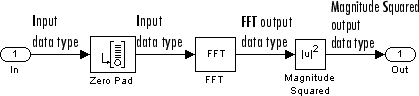Documentation

### This is machine translation

Mouseover text to see original. Click the button below to return to the English version of the page.

# Magnitude FFT

Compute nonparametric estimate of spectrum using periodogram method

## Library

• Estimation / Power Spectrum Estimation

`dspspect3`

• Transforms

`dspxfrm3`## Description

The Magnitude FFT block computes a nonparametric estimate of the spectrum using the periodogram method.

When the Output parameter is set to `Magnitude squared`, the block output for an M-by-N input u is equivalent to

`y = abs(fft(u,nfft)).^2 % M ≤ nfft`

When the Output parameter is set to `Magnitude`, the block output for an input u is equivalent to

`y = abs(fft(u,nfft)) % M ≤ nfft`

When M > Nfft, the block wraps the input to Nfft before computing the FFT using one of the above equations:

`y(:,k)=datawrap(u(:,k),nfft) % 1 ≤ k ≤ N`

When M > Nfft, the block can also truncate the input:

`y(:,k)=abs(fft(u,nfft)) % 1 ≤ k ≤ N`

The block treats an M-by-N matrix input as M sequential time samples from N independent channels. The block computes a separate estimate for each of the N independent channels and generates an Nfft-by-N matrix output. Each column of the output matrix contains the estimate of the corresponding input column's power spectral density at Nfft equally spaced frequency points in the range [0,Fs), where Fs represents the signal's sample frequency. The block always outputs sample–based data.

The Magnitude FFT block supports real and complex floating-point inputs. The block also supports real fixed-point inputs in both `Magnitude` and `Magnitude squared` modes, and complex fixed-point inputs in the `Magnitude squared` mode.

### Fixed-Point Data Types

The following diagram shows the data types used within the Magnitude FFT subsystem block for fixed-point signals.The settings for the fixed-point parameters of the FFT block in the diagram above are as follows:

• Sine table — ```Same word length as input```

• Integer rounding mode — `Floor`

• Saturate on integer overflow — unchecked

• Product output — ```Inherit via internal rule```

• Accumulator — ```Inherit via internal rule```

• Output — ```Inherit via internal rule```

The settings for the fixed-point parameters of the Magnitude Squared block in the diagram above are as follows:

• Integer rounding mode — `Floor`

• Saturate on integer overflow — checked

• Output — ```Inherit via internal rule```

## Parameters

Output

Specify whether the block computes the magnitude FFT or magnitude-squared FFT of the input.

FFT implementation

Set this parameter to `FFTW` to support an arbitrary length input signal. The block restricts generated code with FFTW implementation to MATLAB® host computers.

Set this parameter to `Radix-2` for bit-reversed processing, fixed or floating-point data, or for portable C-code generation using the Simulink® Coder™. The first dimension M, of the input matrix must be a power of two. To work with other input sizes, use the Pad block to pad or truncate these dimensions to powers of two, or if possible choose the FFTW algorithm.

Set this parameter to `Auto` to let the block choose the FFT implementation. For non-power-of-two transform lengths, the block restricts generated code to MATLAB host computers.

Inherit FFT length from input dimensions

Select to use the input frame size as the number of data points, on which to perform the FFT. When you select this check box, this number must be a power of two. When you do not select this check box, the FFT length parameter specifies the number of data points.

FFT length

Enter the number of data points on which to perform the FFT, Nfft. When Nfft is larger than the input frame size, each frame is zero-padded as needed. When Nfft is smaller than the input frame size, each frame is wrapped as needed. This parameter is enabled when you clear the Inherit FFT length from input dimensions check box.

When you set the FFT implementation parameter to `Radix-2`, this value must be a power of two.

Wrap input data when FFT length is shorter than input length

Choose to wrap or truncate the input, depending on the FFT length. If this parameter is checked, modulo-length data wrapping occurs before the FFT operation, given FFT length is shorter than the input length. If this property is unchecked, truncation of the input data to the FFT length occurs before the FFT operation. The default is checked.

## Supported Data Types

PortSupported Data Types

Input

• Double-precision floating point

• Single-precision floating point

• Fixed point (signed and unsigned)

• 8-, 16-, and 32-bit signed integers

Output

• Double-precision floating point

• Single-precision floating point

• Fixed point (signed only)

• 8-, 16-, and 32-bit signed integers

 Frigo, M. and S. G. Johnson, “FFTW: An Adaptive Software Architecture for the FFT,”Proceedings of the International Conference on Acoustics, Speech, and Signal Processing, Vol. 3, 1998, pp. 1381-1384.

 Oppenheim, A. V. and R. W. Schafer. Discrete-Time Signal Processing. Englewood Cliffs, NJ: Prentice-Hall, 1989.

 Orfanidis, S. J. Introduction to Signal Processing. Englewood Cliffs, NJ: Prentice-Hall, 1995.

 Proakis, J. and D. Manolakis. Digital Signal Processing. 3rd ed. Englewood Cliffs, NJ: Prentice-Hall, 1996.

Watch now